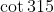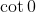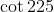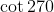Trigonometry : Understand the Signs of the 6 Trigonometric Functions in Each Quadrant

Example Questions

← Previous 1

Example Question #1 : Understand The Signs Of The 6 Trigonometric Functions In Each Quadrant

If the cosine of an angle is negative, but the tangent is positive, what quadrant(s) does the angle lie in?

Explanation:

Tangent is positive in two quadrants: quadrants 1 and 3 (where sine and cosine have the same sign as each other)

Cosine is negative in two quadrants: quadrants 2 and 3 (the left quadrants, where x is negative).

Example Question #2 : Understand The Signs Of The 6 Trigonometric Functions In Each Quadrant

Which of the following is positive?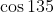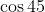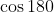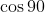Explanation:

When drawn from the origin, a line 45 degrees above (counterclockwise from) the positive x-axis lies in quadrant I. Cosine is defined as the ratio between the adjacent side of a triangle and the hypotenuse of the triangle. A right triangle can be drawn in quadrant I composed of any point on that line, the origin and a point on the x-axis. The hypotenuse of this triangle is considered a length, and is therefore positive. The adjacent side of this triangle lies along the positive x-axis. Since the adjacent side and hypotenuse are both represented by positive numbers, the fraction A/H is positive. Therefore, cos 45 is positive.

Example Question #3 : Understand The Signs Of The 6 Trigonometric Functions In Each Quadrant

Which of the following is positive?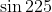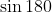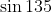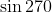Explanation:

When drawn from the origin, a line 135 degrees counterclockwise from the positive x-axis lies in quadrant II. Sine is defined as the ratio between the opposite side of a triangle and the hypotenuse of the triangle. A right triangle can be drawn in quadrant II composed of any point on that line, the origin and a point on the x-axis. The hypotenuse of this triangle is considered a length, and is therefore positive. The opposite side of this triangle lies above the x-axis, and is therefore represented by a positive number. Since the opposite side and hypotenuse are both represented by positive numbers, the fraction O/H is positive. Therefore, sin 135 is positive.

Example Question #4 : Understand The Signs Of The 6 Trigonometric Functions In Each Quadrant

Which of the following is negative?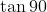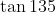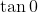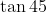Explanation:

When drawn from the origin, a line 135 degrees counterclockwise from the positive x-axis lies in quadrant II. Tangent is defined as the ratio between the opposite and adjacent sides of a triangle. A right triangle can be drawn in quadrant II composed of any point on that line, the origin and a point on the x-axis. The opposite side of this triangle lies above the x-axis, and is therefore represented by a positive number. The adjacent side of this triangle lies on the negative x-axis, and is therefore represented by a negative number. Since the opposite side and adjacent side have opposite signs, the fraction O/A is negative. Therefore, tan 135 is negative.

Example Question #5 : Understand The Signs Of The 6 Trigonometric Functions In Each Quadrant

Which of the following is negative?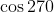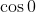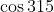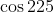Explanation:

When drawn from the origin, a line 225 degrees counterclockwise from the positive x-axis lies in quadrant III. Cosine is defined as the ratio between the adjacent side of a triangle and the hypotenuse of the triangle. A right triangle can be drawn in quadrant III composed of any point on that line, the origin and a point on the x-axis. The hypotenuse of this triangle is considered a length, and is therefore positive. The adjacent side of this triangle lies on the negative x-axis, and is therefore represented by a negative number. Since the opposite side and hypotenuse have opposite signs, the fraction A/H is negative. Therefore, cos 225 is negative.

Example Question #6 : Understand The Signs Of The 6 Trigonometric Functions In Each Quadrant

Which of the following is negative?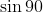Explanation:

When drawn from the origin, a line 225 degrees counterclockwise from the positive x-axis lies in quadrant III. Sine is defined as the ratio between the adjacent side of a triangle and the hypotenuse of the triangle. A right triangle can be drawn in quadrant III composed of any point on that line, the origin and a point on the x-axis. The hypotenuse of this triangle is considered a length, and is therefore positive. The opposite side of this triangle lies below the x-axis, and is therefore represented by a negative number. Since the opposite side and hypotenuse have opposite signs, the fraction O/H is negative. Therefore, sin 225 is negative.

Example Question #7 : Understand The Signs Of The 6 Trigonometric Functions In Each Quadrant

Which of the following is positive?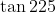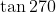Explanation:

When drawn from the origin, a line 225 degrees counterclockwise from the positive x-axis lies in quadrant II. Tangent is defined as the ratio between the opposite and adjacent sides of a triangle. A right triangle can be drawn in quadrant III composed of any point on that line, the origin and a point on the x-axis. The opposite side of this triangle lies below the x-axis, and is therefore represented by a negative number. The adjacent side of this triangle lies on the negative x-axis, and is therefore represented by a negative number. Since the opposite side and adjacent side have the same sign, the fraction O/A is positive. Therefore, tan 225 is positive.

Example Question #8 : Understand The Signs Of The 6 Trigonometric Functions In Each Quadrant

Which of the following is positive?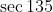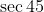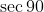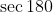Explanation:

When drawn from the origin, a line 45 degrees above (counterclockwise from) the positive x-axis lies in quadrant I. Cosine is defined as the ratio between the adjacent side of a triangle and the hypotenuse of the triangle. Secant is defined as the reciprocal of cosine; therefore, the cosine and secant of an angle have the same sign. A right triangle can be drawn in quadrant I composed of any point on that line, the origin and a point on the x-axis. The hypotenuse of this triangle is considered a length, and is therefore positive. The adjacent side of this triangle lies along the positive x-axis. Since the adjacent side and hypotenuse are both represented by positive numbers, the fraction A/H is positive. Therefore, cos 45 is positive, as is its reciprocal, sec 45.

Example Question #9 : Understand The Signs Of The 6 Trigonometric Functions In Each Quadrant

Which of the following is positive?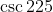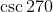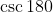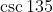Explanation:

When drawn from the origin, a line 135 degrees counterclockwise from the positive x-axis lies in quadrant II. Sine is defined as the ratio between the opposite side of a triangle and the hypotenuse of the triangle. Cosecant is defined as the reciprocal of sine, so the sine and cosecant of an angle must have the same sign. A right triangle can be drawn in quadrant II composed of any point on that line, the origin and a point on the x-axis. The hypotenuse of this triangle is considered a length, and is therefore positive. The opposite side of this triangle lies above the x-axis, and is therefore represented by a positive number. Since the opposite side and hypotenuse are both represented by positive numbers, the fraction O/H is positive. Therefore, sin 135 is positive, as is its reciprocal, csc 135.

Example Question #10 : Understand The Signs Of The 6 Trigonometric Functions In Each Quadrant

Which of the following is positive?